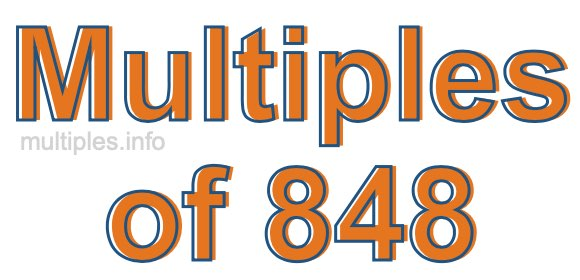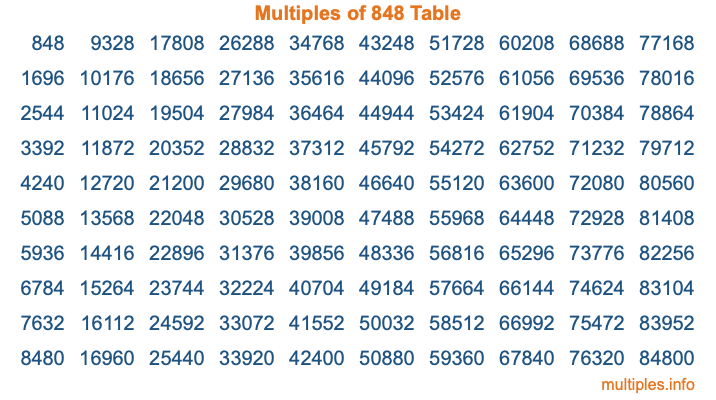Multiples of 848Welcome to the Multiples of 848 page. Here we will first teach you everything you will ever need to know about the multiples of 848, and then give you a study guide summary of everything we taught you to make sure you remember it all. Use this page to look up facts and learn information about the multiples of 848. This page will make you a multiples of eight hundred forty-eight expert!

Definition of Multiples of 848
Multiples of 848 are all the numbers that when divided by 848 equal an integer. Each of the multiples of 848 are called a multiple. A multiple of 848 is created by multiplying 848 by an integer.

Therefore, to create a list of multiples of 848, you start with 1 multiplied by 848, then 2 multiplied by 848, then 3 multiplied by 848, and so on for as long as you want. Thus, the list of the first five multiples of 848 is 848, 1696, 2544, 3392, and 4240. To see a larger list of multiples of 848, see the printable image of Multiples of 848 further down on this page. We also have a category where you can choose any nth multiple of 848.

Multiples of 848 Checker
The Multiples of 848 Checker below checks to see if any number of your choice is a multiple of 848. In other words, it checks to see if there is any number (integer) that when multiplied by 848 will equal your number. To do that, we divide your number by 848. If the the quotient is an integer, then your number is a multiple of 848.

Is  a multiple of 848?

Least Common Multiple of 848 and ...
A Least Common Multiple (LCM) is the lowest multiple that two or more numbers have in common. This is also called the smallest common multiple or lowest common multiple and is useful to know when you are adding our subtracting fractions. Enter one or more numbers below (848 is already entered) to find the LCM.

Check out our LCM Calculator if you need more details about the Least Common Multiple or if you need the LCM for different numbers for adding and subtraction fractions.

nth Multiple of 848
As we stated above, 848 is the first multiple of 848, 1696 is the second multiple of 848, 2544 is the third multiple of 848, and so on. Enter a number below to find the nth multiple of 848.

th multiple of 848

Multiples of 848 vs Factors of 848
848 is a multiple of 848 and a factor of 848, but that is where the similarities end. All postive multiples of 848 are 848 or greater than 848. All positive factors of 848 are 848 or less than 848.

Below is the beginning list of multiples of 848 and the factors of 848 so you can compare:

Multiples of 848: 848, 1696, 2544, 3392, 4240, etc.

Factors of 848: 1, 2, 4, 8, 16, 53, 106, 212, 424, 848

As you can see, the multiples of 848 are all the numbers that you can divide by 848 to get a whole number. The factors of 848, on the other hand, are all the whole numbers that you can multiply by another whole number to get 848.

It's also interesting to note that if a number (x) is a factor of 848, then 848 will also be a multiple of that number (x).

Multiples of 848 vs Divisors of 848
The divisors of 848 are all the integers that 848 can be divided by evenly. Below is a list of the divisors of 848.

Divisors of 848: 1, 2, 4, 8, 16, 53, 106, 212, 424, 848

The interesting thing to note here is that if you take any multiple of 848 and divide it by a divisor of 848, you will see that the quotient is an integer.

Multiples of 848 Table
Below is an image of the first 100 multiples of 848 in a table. The table is in chronological order, column by column. The first column has the first ten multiples of 848, the second column has the next ten multiples of 848, and so on.The Multiples of 848 Table is also referred to as the 848 Times Table or Times Table of 848. You are welcome to print out our table for your studies.

Negative Multiples of 848
Although not often discussed or needed in math, it is worth mentioning that you can make a list of negative multiples of 848 by multiplying 848 by -1, then by -2, then by -3, and so on, to get the following list of negative multiples of 848:

-848, -1696, -2544, -3392, -4240, etc.

Multiples of 848 Summary
Below is a summary of important Multiples of 848 facts that we have discussed on this page. To retain the knowledge on this page, we recommend that you read through the summary and explain to yourself or a study partner why they hold true.

There are an infinite number of multiples of 848.

A multiple of 848 divided by 848 will equal a whole number.

848 divided by a factor of 848 equals a divisor of 848.

The nth multiple of 848 is n times 848.

The largest factor of 848 is equal to the first positive multiple of 848.

848 is a multiple of every factor of 848.

848 is a multiple of 848.

A multiple of 848 divided by a divisor of 848 equals an integer.

848 divided by a divisor of 848 equals a factor of 848.

Any integer times 848 will equal a multiple of 848.

Multiples of a Number
Here you can get the multiples of another number, all with the same attention to detail as we did for multiples of 848 on this page.

Multiples of
Multiples of 849
Did you find our page about multiples of eight hundred forty-eight educational? Do you want more knowledge? Check out the multiples of the next number on our list!